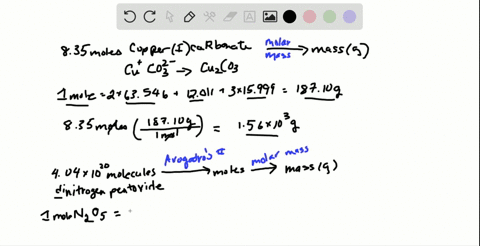🎉 The Study-to-Win Winning Ticket number has been announced! Go to your Tickets dashboard to see if you won! 🎉View Winning Ticket### Calculate each of the following quantities: (a) …

13:49University of Maine
Problem 17

# Calculate each of the following quantities:(a) Total number of ions in 38.1 $\mathrm{g}$ of $\mathrm{SrF}_{2}$(b) Mass $(\mathrm{kg})$ of 3.58 $\mathrm{mol}$ of $\mathrm{CuCl}_{2} \cdot 2 \mathrm{H}_{2} \mathrm{O}$(c) Mass $(\mathrm{mg})$ of $2.88 \times 10^{22}$ formula units of $\mathrm{Bi}\left(\mathrm{NO}_{3}\right)_{3} \cdot 5 \mathrm{H}_{2} \mathrm{O}$

## Discussion

You must be signed in to discuss.

## Video Transcript

When you're given one quantity of a chemical substance, you can change it to another by using the different relationships, including Moeller, Mass. And of a God rose number. It's good to write out a plan to do go through the conversions and then to do the math using dimensional analysis. So if, for example, you know that you have a certain mass of a substance se 33.1 grams of S. R. F two and you know that you want to find out how Maney ions are in that quantity, we always need to start by changing our mass two moles. So we know that we start off with 33 0.1 grams of strong iam fluoride and we change it to moles. We do this by using the molar mass and remember the Moeller masses found by using the atomic number of the different elements found on the periodic table. Once we have moles, we can find out how many formula units and we do this using off a god rose number. And finally, once we have formula units, we can tell how maney ions based on the chemical formula. So if we start off with our initial mass, we should find the molar mass. So to find the molar mass, we use our periodic table and we look at the atomic weights of strong IAM and flooring. Striatum is 87.62 grams per mole, and Florence is 18 0.998 Because there are two florins in the formula, we multiply by two. So the molar mass of strong in Florida is 125 0.62 grams for every one mole. So now we can change our grams, two moles. We know that one mole is equivalent to 125.62 grams. Once we have moles, we changed to formula units using Allah, God rose number 6.2 times 10 to the 23rd for everyone mall and finally for everyone Formula unit. We need to know the number of ions and strong IAM. Fluoride is made up of strong IAM two plus and to Florian Ion. So there are a total of three ion. So we multiply 33.1 times 6.2 times 10 to the 23rd times three and then divide by the molar Mass, which equals 4.76 times, 10 to the 23rd ions. Instead of given Mass, you may be given number of moles to start with 3.58 moves. C u C l two 0.2 h. 20 This is an example of a compound called hydrate, which means that it's made up of copper and to chlorine ions and additionally, to water molecules. So when we find the molar mass, we need to take into account all of those different components, and we want to change it from moles two kilograms. So we know that we start with moles and we want to go to Graham's first, using again the Molar Mass and then from grams two kilograms, simply using the idea that there are 1000 grams for every one kilogram. And so we just need to figure out our Mueller Mass using the periodic table again. So it will be the molar mass of copper, just 63.55 grams, plus two times the molar mass of chlorine just 35 0.45 plus two times two or four times the mass of hydrogen plus two oxygen's which equals 170 point for eight Gramps, we start off with our initial moles to change two grams. We know that one mole is 170 0.48 grams, and once we have grams, we can change two kilograms because one kilogram is 1000 grams so multiplying 3.58 times 170.48 divided by 1000 Z equals two point 610 kilograms. Finally, we can start off with a formula unit or a small particle such as 2.88 times 10 to the 22 and change that to mass or milligrams in this case. So we'll start off with a small particle of formula unit. Change it to malls and the relationship between formula unit and moles is off a god rose number. Once we have moles, we can change mass using Moeller Mass and then finally we can change two milligrams using the relationship between milligrams and grams. There are 1000 milligrams and every one gram. So the only other information we need to know is what the molar masses of the compound and our formula for a compound This is business nitrate and 03 three 0.5 h 20 So it's another hydrate, which means we have to include the mass of the water, and so one mole will have the mass of the massive bismuth from the periodic table. Just 208 0.9 NATO plus three nitrogen three times 14.7 plus three times three or nine oxygen's 99 plus five times two or 10 hydrogen plus five Oxygen's or this compound has a molar mass of 485 0.67 grams. So we start off. There are initial quantity given 2.88 times 10 to the 22nd formula unit changing it two moles. One mole is equal to 6.2 times 10 to the 23rd units, and then we can change it to grams 485.67 grams for every mall. And then finally, we can change two milligrams 1000 milligrams for one grand. So multiplying 2.88 times 10 to the 22nd I was 4.8 485.67 times 1000 divided by 6.2 times 10 to the 23rd you says at 1.5 weaves us it 2.32 times 10 22nd right turned to the fifth milligrams.